## Sunday, December 30, 2007

### Roots of Unity

For n ≥ 3, xn = 1 has three or more distinct roots. While (1, -1) were well known during Abraham de Moivre's day. The other roots were not so well known. It took de Moivre's formula to establish these roots on a firm footing. Roger Cotes had independently discovered the properties of the roots of unity as demonstrated by his formulas. Unfortunately, he had died before he could explain many of his discoveries in detail. It was left to de Moivre to explain Cotes' formulas.

In a previous blog, I showed how de Moivre came up with his famous formula based on the work of Francois Viete. In today's blog, I will show how he provided a proof for the existence of the roots of unity.

In 1739, de Moivre used his formula to find the nth root for any complex number (a + bi).

Theorem 1: For any complex number, there are n distinct nth roots.

if a,b ≠ 0, then:where:

0 ≤ k ≤ n-1

Proof:

(1) Let a,b be real numbers such that either a ≠ 0 or b ≠ 0

(2) We note first that: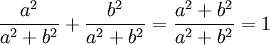(3) Since:It is clear that:And further that:(4) Therefore, there exists φ such that [see here for review of cosine if needed]:(5) Since cos2(φ) + sin2(φ) = 1 (see Corollary 2, here), we can see that:

sin2(φ) = 1 - cos2(φ) =(6) So that we also have now:(7) We can now conclude: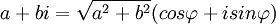since: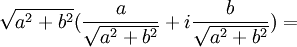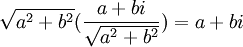(8) Using De Moivre's formula (see Theorem 1, here), we know that:(9) From the basic properties of sin and cosine, we know that:

cos(φ + 2kπ) + isin(φ 2kπ) = cos(φ) + isin(φ)

(10) Multiplying a2 + b2 to both sides gives us: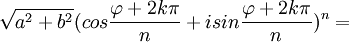(11) Combining step #7 with step #10 gives us:(12) Now, we know that each of the values are distinct for 0 ≤ k ≤ n-1 since none of the angles vary by a multiple of .

QED

Corollary 1.1: Roots of Unity

There are n roots of unity such that: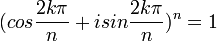where:

0 ≤ k ≤ n-1

Proof:

(1) Let a=1 and b=0

(2) Then a+bi = 1+0*i = 1

(3) Using Theorem 1 above, we have:(4) Using step #4 and step #6 in Theorem 1 above, we have: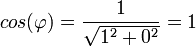and(5) So, φ = 0

(6) Since: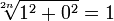we are left with:where:

0 ≤ k ≤ n-1

QED

Corollary 1.2: Roots of -1

There are n roots of unity such that:where:

0 ≤ k ≤ n-1

Proof:

(1) Let a=-1 and b=0

(2) Then a+bi = -1+0*i = -1

(3) Using Theorem 1 above, we have:(4) Using step #4 and step #6 in Theorem 1 above, we have:and(5) So, φ = π

(6) Since: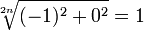we are left with: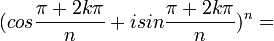where:

0 ≤ k ≤ n-1

QED

References

### De Moivre's Famous Formula

The simple proof of DeMoivre's Formula by Leonhard Euler does not do justice to Abraham de Moivre's important breakthrough on the roots of unity. A person viewing Euler's proof might be surprised that Sir Isaac Newton often answered questions about Principia with: "Go to Mr. de Moivre, he knows these things better than I do."

In today's blog, I will show how de Moivre derived his formula based on Francois Viete's solution of Van Roomen's Problem.

Theorem 1: De Moivre's Formula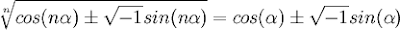Proof:

(1) Let x = 2 cos(α)

(2) Let l = cos(nα)

(3) We can define z such that:(4) So that:

zn = l + √l2 - 1

So that:

zn - l = √l2 - 1

and:

(zn - l)2 = l2 - 1

(5) Working through the equation in step #4, we get:

(zn - l)2 = z2n - 2lzn + l2 = l2 - 1

Subtracting l2 - 1 from both sides gives us:

z2n - 2lzn + 1 = 0

(6) Now, from a Viete's famous solution to Van Roomen's problem (see Corollary 1.1, here), we have:

2 cos(nα) = fn(2 cos α)

where:

fn(x) =(7) Putting step #6 in step #5 gives us:

z2n - 2*(1/2)fn(x)zn + 1 = z2n - fn(x)zn + 1 =0

(8) For n=1, we have:

z2(1) - f1(x)z1 + 1 = 0

(9) Now using the definition for fn(x) in step #6 above, we can see that:

f1(x) = (-1)0(1/[1-0])([1-0]!/0![1 - 2*0]!)x1 - 2*0 =

= 1*(1/1)(1!/[0!1!])x1 =

= x

(10) This then gives us:

z2 - xz + 1 = 0

(11) Now using the quadratic equation to solve for z (see Theorem, here) gives us:

z = (x ± √x2 - 4)/2 =

= x/2 ± √(x/2)2 - 1

(12) Since x = 2cos(α), this gives us:

z = [2 cos(α)]/2 ± √[2 cos(α)/2]2 - 1 =

= cos(α) ± √cos2(α) - 1

(13) Since cos2(x) + sin2(x) = 1 [See Corollary 2, here], we have:

z = cos(α) ± √cos2(α) - 1 =

= cos(α) ± √(-1)[1 - cos2(α)] =

= cos(α) ± √(-1)sin2(α) =

= cos(α) ± [√(-1)]sin(α)

(14) Since l = cos(nα), from step #2 above, we have: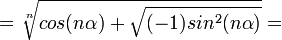(15) Putting step #13 and step #14 together gives us:QED

You might notice that De Moivre's result is ambiguous. Since there are n roots, it is impossible that its result could only be two possible values. This problem was not evident until after there was deeper understanding of the fundamental theorem of algebra. Interestingly, this fundamental theorem was not well understood until after De Moivre's discovery of the roots of unity which I will talk about in my next blog.

The corrected version of de Moivre's formula is:

cos(nα) + isin(nα) = [cos(α) + isin(α)]n

References

## Thursday, December 27, 2007

### Euler's Proof of De Moivre Formula

De Moivre's Formula is easily proven using induction. The following proof was first given by Leonhard Euler in 1748. Abraham De Moivre never stated his formula exactly in this form.

Lemma 1: (cos α + isin α)(cos β + isin β) = cos(α + β) + isin(α + β)

Proof:

(1) cos(α + β) = cos(α)cos(β) - sin(α)sin(β) [See Theorem 2, here]

(2) sin(α + β) = cos(α)sin(β) + cos(β)sin(α) [See Theorem 1, here]

(3) isin(α + β) = cos(α)isin(β) + cos(β)isin(α)

(4) Since isin(α)*isin(β) = -sin(α)sin(β), we have:

cos(α + β) + isin(α + β) =

= cos(α)cos(β) - sin(α)sin(β) + cos(α)isin(β) + cos(β)isin(α) =

= cos(α)[cos(β) + isin(β)] + isin(α)[cos(β) + isin(β)] =

= [cos(α) + isin(α)][cos(β) + isin(β)]

QED

Theorem 1: De Moivre's Formula

(cos α + i sin α)n = cos(nα) + isin(nα) for all α ∈ R.

Proof:

(1) For n=1:

(cos α + i sin α)1 = cos(1*α) + isin(1*α)

(2) Assume that the premise is true for all values up to n such that:

(cos α + i sin α)n = cos(nα) + isin(n&alpha) for all α ∈ R.

(3) (cos α + isin α)n+1 =
(cos α + isin α)n(cos α + isin α)

(4) Using the assumption in step #2, we have:

(cos α + isin α)n(cos α + isin α) =

[cos(n α) + isin(n α)](cos α + isinα)

(5) Using Lemma 1 above, we have:

[cos(n α) + isin(n α)](cos α + isinα) =

cos(n α + α) + isin(nα + α) =

= cos ([n+1]α) + isin([n+1]α)

QED

References

## Friday, December 21, 2007

### Abraham de MoivreAbraham de Moivre was born on May 26, 1667 in Champagne, France. His parents were protestants. When in 1685, Louis XIV overturned the Edict of Nantes which protected the rights of protestants. In this same year, de Moivre was arrested and put in prison for being a protestant. By 1688, he left France for protestant England.

When he was younger, de Moivre had studied classic mathematical works. He had read Christian Huygens text on probability. In the College de Harcourt had taken classes on physics and had studied under the French mathematician Jacques Ozanam. In London, de Moivre became a math tutor.

As a math tutor, de Moivre would teach at the homes of his students or at the London coffee houses. It was at this time that de Moivre learned about Newton's recent book Principia. De Moivre acquired a copy and managed to master it. He did so by removing the pages so he could carry parts of it with him and read it as he had free time.

De Moivre's reputation rose and he soon began meeting the famous mathematicians of England. He became friends with Edmund Halley and Sir Isaac Newton. He presented a paper to the Royal Society in 1695 and became a fellow of the Royal Society in 1697.

In 1710, he served on the committee that investigated the claims of Newton and Leibniz to be the inventors of calculus. Not surprisingly, this English committee ruled in favor of Newton.

De Moivre made many advances in the mathematics of his day. He made important progress in analytic geometry and probability theory. He wrote a textbook on probability in 1718. He did important work on mortality statistics and the study of annuities. Stirling's formula (which should perhaps be properly named De Moivre's formula) first appears in a work by De Moivre in 1733. The famous De Moivre formula never appears directly in any of his work but it is clearly spelled out in a paper in 1722.

Despite his impressive work, de Moivre was unable to find a permanent post and continued to earn his income as a math tutor. As such, he never rose to the same level of wealth as his mathematical peers. While England gave him free reign to be a protestant, it still discriminated against him for being French. De Moivre stayed poor throughout his life and never married.

Legend has it that de Moivre was able to correctly predict the date of his death. Supposedly, he noticed that he was sleeping 15 minutes longer each night. Based on his current duration of sleep, he predicted that he would sleep for 24 hours starting on November 27, 1754.

References

### Roger Cotes' Formulas

Roger Cotes thoroughly investigated the factorization of x4 + a4. In doing so, he found the following formulas which he presented without proof:

Formula 1:Formula 2: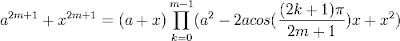Formula 3:Formula 4: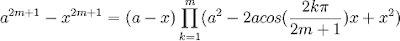These formulas demonstrated that Leibniz's supposition about x4 + a4 was incorrect.

The proof for these formulas would later be provided by Abraham de Moivre as he investigated the properties of the roots of unity.

References: